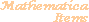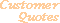Home Older Mathematica Items

Scientific Arts

# Exploring a Data Set: Plotting with Error Bars

## The Data Set

At the URL http://pdg.lbl.gov/~sbl/pipp_total.dat there is a data set that describes the total interaction cross section for the elementary particle reaction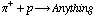. After downloading the file (named pipp_total.dat) from the web we can read it into Mathematica using the Import function. (If the file pipp_total.dat is not placed in a directory that is contained in Mathematica's \$Path variable, you can make it available by executing the function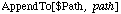where path is the path to the needed directory.)

``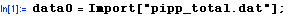``

The first 11 lines of this data set consists of an informational header, the remaining lines contain data. The fifth row names the data that are in each column (there are 11 columns). The columns in the data set are as follows:

``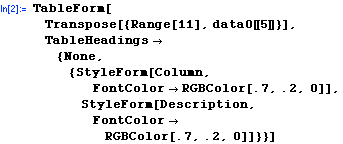``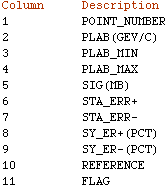From this we see that, column 2 contains the laboratory beam energy, column 5 contains the cross section, and columns 6 and 7 contain the errors. First we drop the header information from the data

``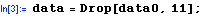``

And now, using pattern matching and transformation rules, we create data sets with the desired information. The list dataWithErrors contains the data along with the error information in the form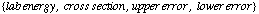and the list dataWithoutErrors contains just the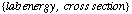data.

``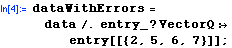``
``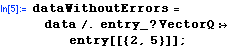``

## Plotting the data

First we make a basic plot of the data.

``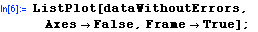``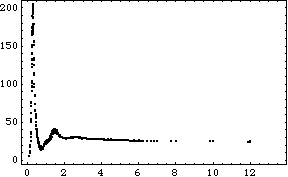There are, in fact, data beyond 14 GeV/c laboratory energy.

``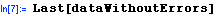``
`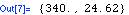`

This data could be seen by choosing the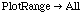option to ListPlot. However, to exhibit the structure of the data, a log-log plot would serve better. Various logarithmic plots are contained in the standard add-on package Graphics`Graphics`. First load the package.

``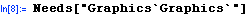``

Now take a look at the data again.

``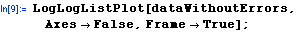``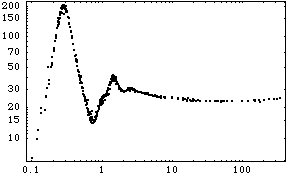Select the data between 1 and 2.5 GeV/c to get a closer look in that region.

``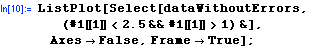``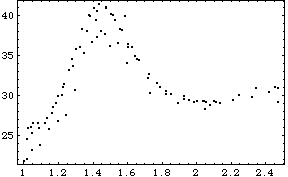## Plotting with Error Bars: MultipleListPlot

One way that we can put errors onto this plot is to make use of the standard add-on package Graphics`MultipleListPlot`.

 There are several ways to specify the points in the data sets used by MultipleListPlot. The most basic involve only the locations of the points. If a point is given as a single number, the value is treated as a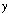value, and the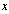value is determined by the position of the point in the list of data. If given as a pair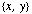, the point is placed at thecoordinates. Each point can be grouped with an error specification, given as an ErrorBar object. An ErrorBar object can accept a specification of error in thevariable or in bothand. An error given as a single value indicates the same error in the positive and negative directions; a negative number paired with a positive number indicates a different error in each direction.

``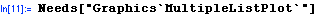``

Here is the usage message for ErrorBar:

``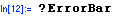``
`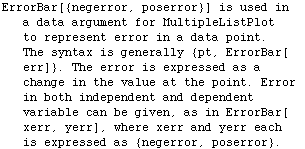`

The selected data can be put into this form through a transformation rule.

``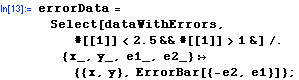``
``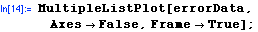``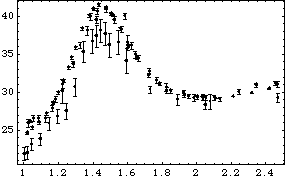It appears from looking at this plot that, of the various data sources that were combined to produce this overall data set, there appear to be some (perhaps one?) that have statistics that are quite different from the others. One could continue to investigate this by partitioning the data according to the "Reference" field to discover whether this hypothesis is true.

## Plotting with Errors: Graphics Primitives

Lets take a different approach to producing this plot. Here we will work with graphics primitives to gain more control over the form that this plot takes (although Graphics`MultipleListPlot` does give you more control than we have used above), and to ultimately produce a customized graphic.

Select the data between 1 and 2.5 GeV/c again

``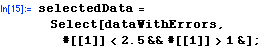``

Our approach will be to convert the data of the form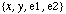into graphics directives: Points and Lines. Thus, for each data point to have error lines extending above and below, we would want something of the form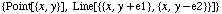. We can do this easily with a transformation rule.

``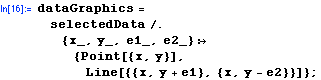``

Display the graphics primitives by enclosing them in Graphics and then use Show.

``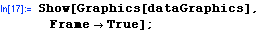``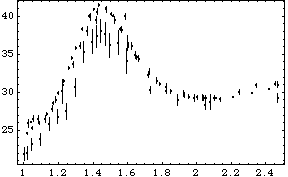Let's color the error bars red and make the data points larger.

``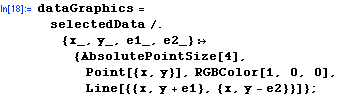``
``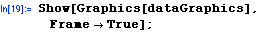``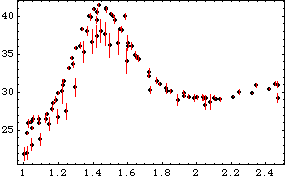Now we color the error bars blue when either of the errors is greater than 0.5

``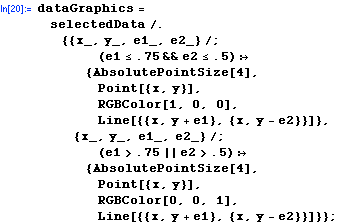``
``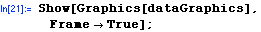``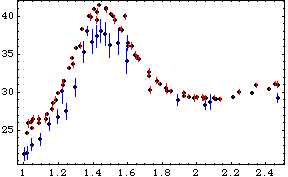This sort of graphics development and exploration can be continued along these lines so as to customize a set of data visualization tools for your specific needs. (A simple extension of this example would be to add the serifs on the tops and bottoms of the error bars: this just involves the addition of some more line primitives to the transformation rules used here.) You could then gather the resulting code together to produce a function that can be reused as needed. Here is a basic example that makes use of what we have done here.

``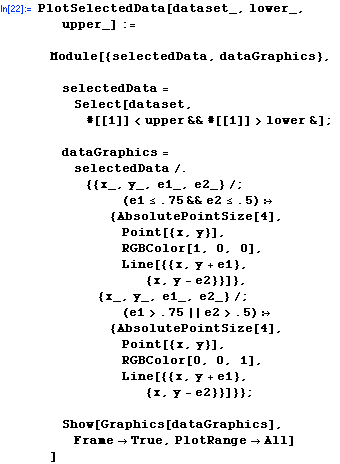``

Here is an example of its use.

``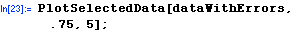``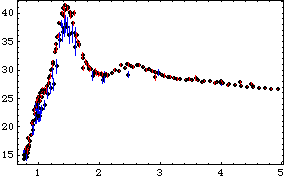For further information on our services send email to info@scientificarts.com . Contents of this web site Copyright © 1999-2011, Scientific Arts, LLC.
 s